### Home > CAAC > Chapter 13 > Lesson 13.IF2-S > Problem6-33

6-33.
1.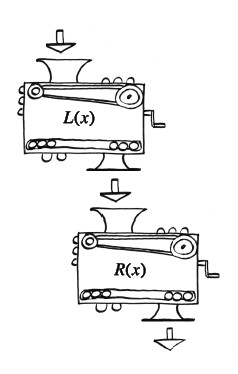Lacey and Richens each have their own personal function machines. Lacey's, L(x), squares the input and then subtracts one. Richen's function, R(x), adds 2 to the input and then multiplies the result by three. Homework Help ✎

1. Write the equations that represent L(x) and R(x).

2. Lacey and Richens decide to connect their two machines, so that Lacey's output becomes Richens' input. Eventually, what is the output if 3 is the initial input?

3. What if the order of the machines was changed? Would it change the output? Justify your answer.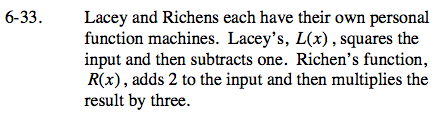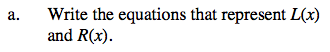L(x) = x2 − 1

R(x) = 3(x + 2)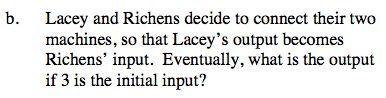30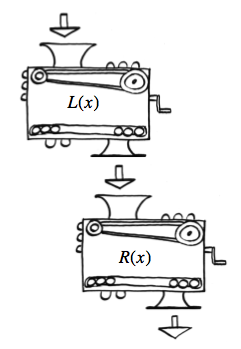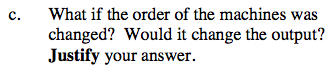Try computing the result for x = 3 if the order is reversed.